Do you like the article?
Share it with others -

Use new possibilities of MetaTrader 5

# Creating Multi-Colored Indicators in MQL5

2 August 2010, 15:30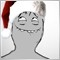1
15 049

### Introduction

Thanks to the efforts of MetaTrader 5 developers, the MQL5 language has appeared. There are a lot of innovations, but here I will describe and consider the possibility of creation of multi-color indicators. In MQL4 the color can be specified for a line, it's the same for the whole line, and the multi-color indicators are created using the partial overlap of the indicator's buffers, which is not convenient.

The developers of the MQL5 language have provided a new possibility - to specify a color for each section of the indicator's line (for lines) and colors of separate objects (for bars, candles, histograms, arrows). To understand this article, it's better to have a look at MQL5 Reference.

• Basics of indicators
• Data buffers of the indicator
• Color index buffers of the indicator
• How to convert one-color drawing mode to multi-color on the example of the RSI indicator (conversion of DRAW_LINE to DRAW_COLOR_LINE drawing styles)
• How to paint the candlestick chart (using the DRAW_COLOR_CANDLES drawing style) depending on the values of the RSI indicator
• How to get the value from the buffer of color indexes
Let's consider two color drawing styles - DRAW_COLOR_LINE and DRAW_COLOR_CANDLES, remaining drawing styles differ only in the number of buffers.

### Why color indicators?

Using the color indicators, you will be able to:

• Show additional information on candles.
• Create hybrids of indicators (the MACD color depends on the values of RSI).
• Highlight the important signals of the indicator.
• Just simply decorate your client terminal.

### The MQL5 basics

Generally, the indicator gets input data (prices, the other indicator's data), performs some calculations and fills several buffers with the data. The client terminal plots the information from the buffers, provided by the indicator according to its drawing type.

The drawing style is defined by the developer. The indicator buffers are arrays of double type, declared at the global level. Several buffers can be combined into the graphic plots, if more than one buffer is needed for a style. If you have never created custom indicators, you may read the articles (the basics are well described there): "MQL5: Create Your Own Indicator" and "Custom Indicators in MQL5 for Newbies".

Here is the minimal code of the color indicator, I will describe its components:

```//+------------------------------------------------------------------+
//|                                         test_color_indicator.mq5 |
//|                                                             ProF |
//|                                                          http:// |
//+------------------------------------------------------------------+
#property indicator_separate_window             //The indicator is plotted in a separate window
#property indicator_buffers 2                   //Number of indicator buffers
#property indicator_plots 1                     //Number of indicator plots
#property indicator_type1 DRAW_COLOR_HISTOGRAM  //Drawing style - Color Histogram
#property indicator_width1 3                    //Line width of a graphic plot (optional)
#property indicator_color1 Red,Green,BlueViolet //Specify 3 colors for a graphic plot

//Declaration of buffers
double buffer_line[]/*Data Buffer*/, buffer_color_line[]/*Color index buffer*/;

//+------------------------------------------------------------------+
//| Custom indicator initialization function                         |
//+------------------------------------------------------------------+
int OnInit()
{
//Assign the data array with indicator's buffer
SetIndexBuffer(0,buffer_line,INDICATOR_DATA);

//Assign the color indexes array with indicator's buffer
SetIndexBuffer(1,buffer_color_line,INDICATOR_COLOR_INDEX);

//Specify the number of color indexes, used in the graphic plot
PlotIndexSetInteger(0,PLOT_COLOR_INDEXES,2);

//Specify colors for each index
PlotIndexSetInteger(0,PLOT_LINE_COLOR,0,Blue);   //Zeroth index -> Blue
PlotIndexSetInteger(0,PLOT_LINE_COLOR,1,Orange); //First index  -> Orange
return(0);
}
//+------------------------------------------------------------------+
//| Custom indicator iteration function                              |
//+------------------------------------------------------------------+
int OnCalculate(const int rates_total,
const int prev_calculated,
const datetime &time[],
const double &open[],
const double &high[],
const double &low[],
const double &close[],
const long &tick_volume[],
const long &volume[],
{
//For each bar we fill the data buffer and index color buffer with values
for(int i=prev_calculated;i<=rates_total-1;i++)
{
//Lets add a simple drawing condition -> If opening price is greater than closing price, then:
if(open[i]>close[i])
{   buffer_color_line[i]=0;   }       //Assign color with index=zero (0)
else
{  buffer_color_line[i]=1;   }       //Assign color with index=one (1)

//Specify the data for plotting, in our case it's the opening price
buffer_line[i]=open[i];
}

return(rates_total-1); //Return the number of calculated bars,
//subtract 1 for the last bar recalculation
}
//+------------------------------------------------------------------+
```

Let us examine the details of writing the color indicators:

```#property indicator_buffers 2 //Number of indicator's buffers
#property indicator_plots 1   //Number of graphic plots```

In the first line we specify the number of indicator buffers, in our case we have two buffers:

1. The buffer for the indicator data, in our case, for the opening prices;
2. The buffer for the color indexes.

In the second line, we specify the number of graphics. It's important to distinguish the graphics and the indicator's buffers. The graphics is the line (candle, bar, arrow, etc.) of the indicator. An indicator buffer is an array with data, needed to plot, array with color indexes or an array for the internal calculations of the indicator (this type is not drawn in the indicator's window).

The number of plots may be equal or less than the number of buffers, it depends on the drawing style and number of buffers for the calculation. The table with drawing styles and number of buffers needed for each style is available in the Drawing Styles chapter of MQL5 Reference.

The "most interesting" begins here:

```#property indicator_type1 DRAW_COLOR_HISTOGRAM  //Drawing style-color histogram
#property indicator_width1 3                    //Drawing line width (optional)
#property indicator_color1 Red,Green,BlueViolet //Specify 3 colors for a graphic plot```

In the first line, we specify the drawing style, in our case the drawing style is a histogram from a zero line. This drawing style requires a data buffer and a color index buffer. All drawing styles containing the word "COLOR" require a buffer for color indexes.

In the second line, we specify the width of a line equal to three pixels, by default, the width of a line is set to one pixel.

In the third line, we specify the colors for the indexes of the graphics, in this case, we specified three colors "Red", "Green" and "BlueViolet". The color indexes are starting from zero: 0-"Red", 1-"Green", 2-"BlueViolet". The colors are required for setting colors of the graphics. The colors can be specified in several ways, the "#property indicator_color1" is one of them. This is a "static" method, it is used at the stage of the program compilation. The second method is discussed below.

```double buffer_line[]/*Data buffer*/, buffer_color_line[]/*Color indexes buffer*/;
```

Here we declare two arrays that will be used as buffers, the first will be used as a data buffer, the second will be used for the color indexes, both declared as the arrays of double type.

Let's consider the indicator initialization function:

`SetIndexBuffer(0,buffer_line,INDICATOR_DATA);`

Here we assign the indicator buffer with an array, the specified "INDICATOR_DATA" buffer type means that this buffer will be used to store the values of the indicator (i.e. it's the data buffer of the indicator). Note, that the first parameter is equal to zero (0) - it's the buffer index.

`SetIndexBuffer(1,buffer_color_line,INDICATOR_COLOR_INDEX);`

Here we assign the indicator buffer with an array and specify "INDICATOR_COLOR_INDEX" as the buffer type - it means that this buffer will be used to store the color indexes for each bar of the indicator. Note that the first parameter is equal to (1), it's the buffer index.

The buffer ordering must be special: first of all, the indicator data buffers, then the color index buffers.

And finally, the second way to specify the colors of the graphics (to specify the color indexes):

```//Specify the number of color indexes, used in the graphics
PlotIndexSetInteger(0,PLOT_COLOR_INDEXES,2);```

Here we specify the number of color indexes. The first parameter of the function is equal to "0", it's the graphics index. Note that in this case we must specify the number of color indexes (in the first method, the compiler calculates it).

```PlotIndexSetInteger(0,PLOT_LINE_COLOR,0,Blue);   //Zeroth index -> Blue
PlotIndexSetInteger(0,PLOT_LINE_COLOR,1,Orange); //First index  -> Orange
```

Here we specify the colors for each index. The first parameter of the function is the index of the graphics, the third parameter is the color index, starting from zero. The second way of setting color indexes differs in the following: the number of colors and their indexes can be specified dynamically, for example, using the function. If you use both methods, remember that the dynamic method overrides the static (first method).

Next, consider the OnCalculate function, we calculate the buffer values for the indicator's graphics. We choose the simplest rules for the color selection for the histogram, if the open price is greater than  the close price, we assign the current buffer element with the color index (in the "buffer_color_line" array) equal to zero (0). The color index, equal to zero (0) corresponds to the "Blue" color, specified above.

If the open price is lower than the close price, we assign the color index, equal to 1, that corresponds to Orange color. Here is this simple example: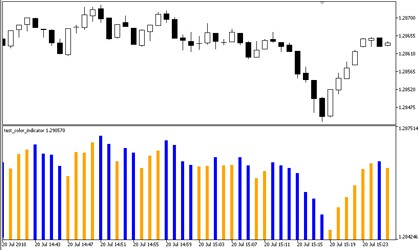One can see, it's easy, we only need some imagination.

### The Methods of Color Setting

Now, let's consider the details of color setting.

According to MQL5 Reference, the color can be specified using different methods:

• Literally;
• Numerically;
• Using color names.

Let's consider all of them.

Literally

```color color_var = C'10,20,255';
color color_var = C'0x0A,0x14,0xFF';```

The colors are defined according to the RGB (Red, Green, Blue), any color can be presented as a sum of these three colors. Accordingly, the first number corresponds to Red color component. The second corresponds to Green, the third corresponds to the Blue component. The numbers (in decimal form) can be from 0 to 255. In hexadecimal base, the values can be from 00 to FF.

The first and the second lines are equal: we assign Blue color to the color_var variable. The difference is the representation of a number in specified numeral systems, the decimal in the first line, and hexadecimal in the second line. There isn't any difference, you can choose the way, convenient for you. The smaller number corresponds to the darker colors, the white color is: "C'255,255,255'" or "C'0xFF,0xFF,0xFF'", the black color is: "C'0,0,0'" or "C'0x00,0x00,0x00'".

Numerically

```color color_var = 0xFFFFFF;  // white
color color_var = 0x0000FF;  // red
color color_var = 16777215   // white
color color_var = 0x008000   // green
color color_var = 32768      // green```

The colors are represented in hexadecimal and decimal numeral systems. For example, the value "0x0000FF" is equal to "C'0xFF,0x00,0x00'", as we see, the first and the last pairs of the numbers are swapped.

To get the value 16777215 in the decimal numerical system, we need to convert the number FFFFFF from hexadecimal to the decimal numeral system.

Color names

```color color_var = Red;    //red
color color_var = Blue;   //blue
color color_var = Orange; //orange```

This is the simplest way, but you can specify only colors from the web-colors set.

Let's summarize how we can specify colors.

All the three methods are equal, for example:

```color color1 = C'255,0,0';
color color2 = C'0xFF,0x00,0x00';
color color3 = 0x0000FF;
color color4 = 255;
color color5 = Red;

&& (color1==color2)
&& (color1==color4)
&& (color1==color5)); //prints true
```

### A Practice

We have learned the basics, now let's consider how to paint the chart candles with different colors, depending on the other indicator values, for example, depending on RSI values. To create the color candlesticks on the chart, we need to write an indicator that will plot the imposed color candles on the chart.

Here is the code of the indicator, if the values of RSI are less than 50%, it plots Blue candles, otherwise the candles are plotted with Orange color.

To avoid the confusion of a reader, there isn't checking for the correctness of the data and error processing. But these details should be taken into account when writing the working code of the indicator.

```//+------------------------------------------------------------------+
//|                                                   cand_color.mq5 |
//|                                                             ProF |
//|                                                          http:// |
//+------------------------------------------------------------------+
#property indicator_chart_window                //Indicator in separate window

//Specify the number of buffers of the indicator
//4 buffer for candles + 1 color buffer + 1 buffer to serve the RSI data
#property indicator_buffers 6

//Specify the names in the Data Window
#property indicator_label1 "Open;High;Low;Close"

#property indicator_plots 1                     //Number of graphic plots
#property indicator_type1 DRAW_COLOR_CANDLES    //Drawing style - color candles
#property indicator_width1 3                    //Width of the graphic plot (optional)

//Declaration of buffers
double buffer_open[],buffer_high[],buffer_low[],buffer_close[]; //Buffers for data
double buffer_color_line[];    //Buffer for color indexes
double buffer_tmp;           //Temporary buffer for RSI data copying
double buffer_RSI[];            //Indicator buffer for RSI
int handle_rsi=0;               //Handle for the RSI indicator
//+------------------------------------------------------------------+
//| Custom indicator initialization function                         |
//+------------------------------------------------------------------+
int OnInit()
{
/**
*       The order of the buffers assign is VERY IMPORTANT!
*  The data buffers are first
*       The color buffers are next
*       And finally, the buffers for the internal calculations.
*/
//Assign the arrays with the indicator's buffers
SetIndexBuffer(0,buffer_open,INDICATOR_DATA);
SetIndexBuffer(1,buffer_high,INDICATOR_DATA);
SetIndexBuffer(2,buffer_low,INDICATOR_DATA);
SetIndexBuffer(3,buffer_close,INDICATOR_DATA);

//Assign the array with color indexes with the indicator's color indexes buffer
SetIndexBuffer(4,buffer_color_line,INDICATOR_COLOR_INDEX);

//Assign the array with the RSI indicator data buffer
SetIndexBuffer(5,buffer_RSI,INDICATOR_CALCULATIONS);

//Define the number of color indexes, used for a graphic plot
PlotIndexSetInteger(0,PLOT_COLOR_INDEXES,2);

//Set color for each index
PlotIndexSetInteger(0,PLOT_LINE_COLOR,0,Blue);   //Zeroth index -> Blue
PlotIndexSetInteger(0,PLOT_LINE_COLOR,1,Orange); //First index  -> Orande

//Get handle of RSI indicator, it's necessary to get the RSI indicator values
handle_rsi=iCustom(_Symbol,_Period,"Examples\\RSI");
return(0);
}
//+------------------------------------------------------------------+
//| Custom indicator iteration function                              |
//+------------------------------------------------------------------+
int OnCalculate(const int rates_total,
const int prev_calculated,
const datetime &time[],
const double &open[],
const double &high[],
const double &low[],
const double &close[],
const long &tick_volume[],
const long &volume[],
{
//In the loop we fill the data buffers and color indexes buffers for each bar
for(int i=prev_calculated;i<=rates_total-1;i++)
{
//Copying the RSI indicator's data to the temporary buffer - buffer_tmp
CopyBuffer(handle_rsi,0,BarsCalculated(handle_rsi)-i-1,1,buffer_tmp);
//Copying the values from the temporary buffer to the indicator's buffer
buffer_RSI[i]=buffer_tmp;

//Set data for plotting
buffer_open[i]=open[i];  //Open price
buffer_high[i]=high[i];  //High price
buffer_low[i]=low[i];    //Low price
buffer_close[i]=close[i];//Close price

//Add a simple condition -> If RSI less 50%:
if(buffer_RSI[i]<50)
{   buffer_color_line[i]=0;   } //Assign the bar with color index, equal to 0
else
{  buffer_color_line[i]=1;   }  //Assign the bar with color index, equal to 1
}
return(rates_total-1); //Return the number of calculated bars,
//Subtract 1 for the last bar recalculation
}
//+------------------------------------------------------------------+```

Here is how it looks like: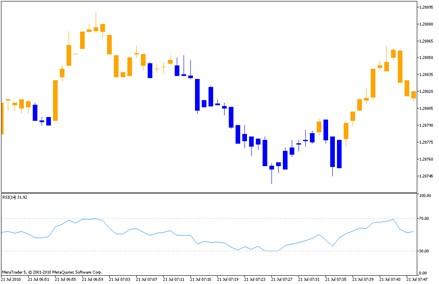It looks good, but we will go ahead.

Let's paint the candles depending on the values of RSI using many colors, so called gradient filling.

The colors can be specified manually, but it isn't convenient and easy to specify 30-40 colors. We will do the following: we will write two functions, the first for the color indexes, the second is for getting the color depending on arguments of the function. The idea is written in comments.

```//+------------------------------------------------------------------+
//|                                               cand_color_RSI.mq5 |
//|                                                             ProF |
//|                                                          http:// |
//+------------------------------------------------------------------+
#property indicator_chart_window                //Indicator in a separate window

//Specify the number of indicator's buffers
//4 buffers for candles + 1 buffer for color indexes + 1 buffer to store the data of RSI
#property indicator_buffers 6

//Specify the names, shown in the Data Window
#property indicator_label1 "Open;High;Low;Close"

#property indicator_plots 1                     //Number of graphic plots
#property indicator_type1 DRAW_COLOR_CANDLES    //Drawing style - colored candles
#property indicator_width1 3                    //Width of a line (optional)

//Declaration of buffers
double buffer_open[],buffer_high[],buffer_low[],buffer_close[];//Buffers for data
double buffer_color_line[];     //Buffer for color indexes
double buffer_tmp;          //Temporary buffer for RSI values
double buffer_RSI[];            //Indicator buffer for RSI
int handle_rsi=0;               //Handle of the RSI indicator
//+------------------------------------------------------------------+
//|    Set colors for a graphic plot                          |
//+------------------------------------------------------------------+
/*
*       The function sets colors for a graphic plot
*       50 colors from Green to Blue.
*       The index of a graphic plot is passed to the function.

void setPlotColor(int plot)
{
PlotIndexSetInteger(plot,PLOT_COLOR_INDEXES,50); //Specify the number of colors

//In the loops we specify the colors
for(int i=0;i<=24;i++)
{
PlotIndexSetInteger(plot,PLOT_LINE_COLOR,i,StringToColor("\"0,175,"+IntegerToString(i*7)+"\""));
}
for(int i=0;i<=24;i++)
{
PlotIndexSetInteger(plot,PLOT_LINE_COLOR,i+25,StringToColor("\"0,"+IntegerToString(175-i*7)+",175\""));
}
}
//+------------------------------------------------------------------+
//|  Get index of the color                                          |
//+------------------------------------------------------------------+
/*
*       The function returns the index of the color
*       The first parameter is the current value of the indicator
*       The second parameter is the minimal value of the indicator
*       The third parameter is the maximal value of the indicator
*/
int getPlotColor(double current,double min,double max)
{
return((int)NormalizeDouble((50/(max-min))*current,0));
}
//+------------------------------------------------------------------+
//| Custom indicator initialization function                         |
//+------------------------------------------------------------------+
int OnInit()
{
/**
*       The order of the indicator's buffers is VERY IMPORTANT!
*  The data buffers are first
*       The color buffers are next
*       And finally, the buffers for the internal calculations.
*/
//Assign the arrays with the indicator buffers
SetIndexBuffer(0,buffer_open,INDICATOR_DATA);
SetIndexBuffer(1,buffer_high,INDICATOR_DATA);
SetIndexBuffer(2,buffer_low,INDICATOR_DATA);
SetIndexBuffer(3,buffer_close,INDICATOR_DATA);

//Assign the array with the color indexes buffer
SetIndexBuffer(4,buffer_color_line,INDICATOR_COLOR_INDEX);

//Assign the array with RSI indicator buffer
SetIndexBuffer(5,buffer_RSI,INDICATOR_CALCULATIONS);

//Specify color indexes
setPlotColor(0);

//Get handle of the RSI indicator, it's necessary get its values
handle_rsi=iCustom(_Symbol,_Period,"Examples\\RSI",6);
return(0);
}
//+------------------------------------------------------------------+
//| Custom indicator iteration function                              |
//+------------------------------------------------------------------+
int OnCalculate(const int rates_total,
const int prev_calculated,
const datetime &time[],
const double &open[],
const double &high[],
const double &low[],
const double &close[],
const long &tick_volume[],
const long &volume[],
{
//For each bar we fill the data buffers and buffer with color indexes using the loop
for(int i=prev_calculated;i<=rates_total-1;i++)
{
//Copying the RSI indicator data to the temporary buffer buffer_tmp
CopyBuffer(handle_rsi,0,BarsCalculated(handle_rsi)-i-1,1,buffer_tmp);
//Then copying the data from the temporary buffer
buffer_RSI[i]=buffer_tmp;

//Specify the data for plotting
buffer_open[i]=open[i];  //Open price
buffer_high[i]=high[i];  //High price
buffer_low[i]=low[i];    //Low price
buffer_close[i]=close[i];//Close price

//Paint the candles depending on RSI indicator values
//RSI = 0     - candle is Green
//RSI = 100   - candle is Blue
//0<RSI<100   - candle color is between Green and Blue
buffer_color_line[i]=getPlotColor(buffer_RSI[i],0,100);

}
return(rates_total-1); //Return the number of calculated bars,
//Subtract 1 for the last bar recalculation
}
//+------------------------------------------------------------------+
```

Here is how it looks like: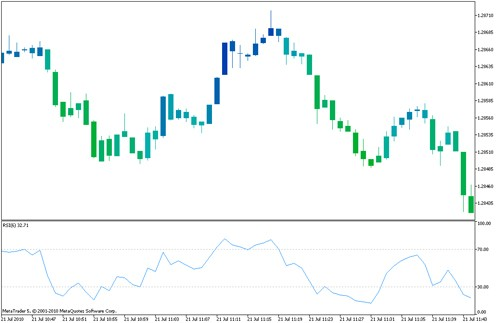Using it as an example, set other colors. Try to replace RSI with another indicator.

The practice is always important.

### Drawing Styles: Conventional and Multi-Color

You can paint an existing indicator, you need to do the following things: change the drawing style to multicolor, add buffers, assign them with the indicator buffers and specify the painting details.

Here is a table of conventional drawing styles and corresponding multi-color (painted) drawing styles:

Before
After
DRAW_LINEDRAW_COLOR_LINE
DRAW_SECTIONDRAW_COLOR_SECTION
DRAW_HISTOGRAMDRAW_COLOR_HISTOGRAM
DRAW_HISTOGRAM2DRAW_COLOR_HISTOGRAM2
DRAW_ARROWDRAW_COLOR_ARROW
DRAW_ZIGZAGDRAW_COLOR_ZIGZAG (example)
DRAW_CANDLESDRAW_COLOR_CANDLES

Here is the code of the modified RSI, painted depending on its own values.

All modifications are commented.

```//+------------------------------------------------------------------+
//|                                                          RSI.mq5 |
//|                        Copyright 2009, MetaQuotes Software Corp. |
//|                                              http://www.mql5.com |
//+------------------------------------------------------------------+
#property copyright   "2009, MetaQuotes Software Corp."
#property description "Relative Strength Index"
//--- indicator settings
#property indicator_separate_window
#property indicator_minimum 0
#property indicator_maximum 100
#property indicator_level1 30
#property indicator_level2 70
/////////////////////////////////////////////////////////////////////
#property indicator_buffers 4 //The number of buffers has increased by 1
#property indicator_width1 5  //The line width has set to 4 pixels
/////////////////////////////////////////////////////////////////////
#property indicator_plots   1
/////////////////////////////////////////////////////////////////////
//Drawing style has been changed from DRAW_LINE to DRAW_COLOR_LINE
#property indicator_type1   DRAW_COLOR_LINE
/////////////////////////////////////////////////////////////////////
#property indicator_color1  DodgerBlue
//--- input parameters
input int InpPeriodRSI=14; // Period
//--- indicator buffers
double    ExtRSIBuffer[];
double    ExtPosBuffer[];
double    ExtNegBuffer[];
//--- global variable
int       ExtPeriodRSI;

//////////////////////////////////////////////////////////////////////
double buffer_color[]; //Declare an array for color indexes

//+------------------------------------------------------------------+
//|    Set color for a graphic plot                                  |
//+------------------------------------------------------------------+
/*
*       The function specify the color for a graphic plot
*       50 colors from Green to Blue are available.
*       The index of a graphic plot is passed to the function.
*/
void setPlotColor(int plot)
{
PlotIndexSetInteger(plot,PLOT_COLOR_INDEXES,50); //Set number of colors

//Specify colors in loop
for(int i=0;i<=24;i++)
{
PlotIndexSetInteger(plot,PLOT_LINE_COLOR,i,StringToColor("\"0,175,"+IntegerToString(i*7)+"\""));
}
for(int i=0;i<=24;i++)
{
PlotIndexSetInteger(plot,PLOT_LINE_COLOR,i+25,StringToColor("\"0,"+IntegerToString(175-i*7)+",175\""));
}
}
//+------------------------------------------------------------------+
//|  Get index of the color                                           |
//+------------------------------------------------------------------+
/*
*       The function returns the color index
*       The first parameter is the current value of the indicator
*       The second parameter is the minimal value of the indicator
*       The third parameter is the maximal value of the indicator
*/
int getPlotColor(double current,double min,double max)
{
return((int)NormalizeDouble((50/(max-min))*current,0));
}
//////////////////////////////////////////////////////////////////////

//+------------------------------------------------------------------+
//| Custom indicator initialization function                         |
//+------------------------------------------------------------------+
void OnInit()
{
//--- check for input
if(InpPeriodRSI<1)
{
ExtPeriodRSI=12;
Print("Incorrect value for input variable InpPeriodRSI =",InpPeriodRSI,
"Indicator will use value =",ExtPeriodRSI,"for calculations.");
}
else ExtPeriodRSI=InpPeriodRSI;
//--- indicator buffers mapping
SetIndexBuffer(0,ExtRSIBuffer,INDICATOR_DATA);

/////////////////////////////////////////////////////////////////////
//Assign the array with buffer of color indexes
SetIndexBuffer(1,buffer_color,INDICATOR_COLOR_INDEX);
//The order of buffers is changed!
SetIndexBuffer(2,ExtPosBuffer,INDICATOR_CALCULATIONS);
SetIndexBuffer(3,ExtNegBuffer,INDICATOR_CALCULATIONS);
//Set colors
setPlotColor(0);
/////////////////////////////////////////////////////////////////////

//--- set accuracy
IndicatorSetInteger(INDICATOR_DIGITS,2);
//--- sets first bar from what index will be drawn
PlotIndexSetInteger(0,PLOT_DRAW_BEGIN,ExtPeriodRSI);
//--- name for DataWindow and indicator subwindow label
IndicatorSetString(INDICATOR_SHORTNAME,"RSI("+string(ExtPeriodRSI)+")");
//--- initialization done
}
//+------------------------------------------------------------------+
//| Relative Strength Index                                          |
//+------------------------------------------------------------------+
int OnCalculate(const int rates_total,
const int prev_calculated,
const int begin,
const double &price[])
{
int    i;
double diff;
//--- check for rates count
if(rates_total<=ExtPeriodRSI)
return(0);
//--- preliminary calculations
int pos=prev_calculated-1;
if(pos<=ExtPeriodRSI)
{
//--- first RSIPeriod values of the indicator are not calculated
ExtRSIBuffer=0.0;
ExtPosBuffer=0.0;
ExtNegBuffer=0.0;
double SumP=0.0;
double SumN=0.0;
for(i=1;i<=ExtPeriodRSI;i++)
{
ExtRSIBuffer[i]=0.0;
ExtPosBuffer[i]=0.0;
ExtNegBuffer[i]=0.0;
diff=price[i]-price[i-1];
SumP+=(diff>0?diff:0);
SumN+=(diff<0?-diff:0);
}
//--- calculate first visible value
ExtPosBuffer[ExtPeriodRSI]=SumP/ExtPeriodRSI;
ExtNegBuffer[ExtPeriodRSI]=SumN/ExtPeriodRSI;
ExtRSIBuffer[ExtPeriodRSI]=100.0-(100.0/(1.0+ExtPosBuffer[ExtPeriodRSI]/ExtNegBuffer[ExtPeriodRSI]));
//--- prepare the position value for main calculation
pos=ExtPeriodRSI+1;
}
//--- the main loop of calculations
for(i=pos;i<rates_total;i++)
{
diff=price[i]-price[i-1];
ExtPosBuffer[i]=(ExtPosBuffer[i-1]*(ExtPeriodRSI-1)+(diff>0.0?diff:0.0))/ExtPeriodRSI;
ExtNegBuffer[i]=(ExtNegBuffer[i-1]*(ExtPeriodRSI-1)+(diff<0.0?-diff:0.0))/ExtPeriodRSI;
ExtRSIBuffer[i]=100.0-100.0/(1+ExtPosBuffer[i]/ExtNegBuffer[i]);
/////////////////////////////////////////////////////////////////////
//Paint it
buffer_color[i] = getPlotColor(ExtRSIBuffer[i],0,100);
/////////////////////////////////////////////////////////////////////
}
//--- OnCalculate done. Return new prev_calculated.
return(rates_total);
}
//+------------------------------------------------------------------+
```

Here is it, you may compare the color of the candles and RSI.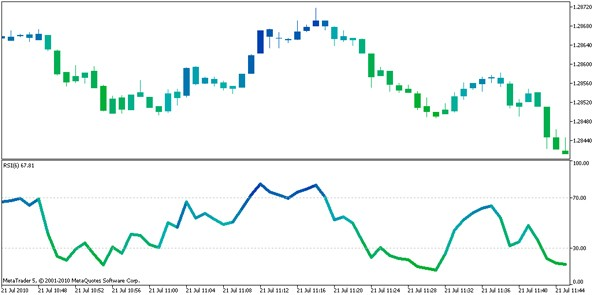### How to Get the Color value of the Indicator from the Expert Advisor/Indicator/Script

Often it's necessary to get the color of a line for automated trading in an Expert Advisor or for some other purposes.

The implementation is simple, let's consider a script.

```//+------------------------------------------------------------------+
//|                                                         test.mq5 |
//|                                                             ProF |
//|                                                          http:// |
//+------------------------------------------------------------------+
#property version   "1.00"
//+------------------------------------------------------------------+
//| Script program start function                                    |
//+------------------------------------------------------------------+
void OnStart()
{
int handle = 0; //handle of the indicator
double tmp;  //temporary array for color index buffer.
//Get the handle of our modified RSI
handle = iCustom(_Symbol,_Period,"Examples\\RSI",6);

//Let's remember, that values are stored in the buffer 1 of our modified RSI
//The color indexes are stored in the buffer 0
//Copying the data from the buffer "1" of the RSI indicator.
CopyBuffer(handle,1,0,1,tmp);

//Show alert with last color index, returned by RSI
Alert(tmp); //For example, if returned 0, it means that RSI
//is painted with Green color and its current level is near 0.
}
//+-----------------------------------------------------------------+```
Note, that we can get the value of the color index, not the color itself!

You must know the correspondence between the color indexes and colors values. In addition, you must know the buffer of color indexes.

To find it out, you need to understand the criteria of the color index setting or determine them empirically by this script or using other methods.

### Conclusion

We have considered the following MQL5 drawing styles: DRAW_COLOR_LINE, DRAW_COLOR_CANDLES. We have painted the candles and learned how to paint the RSI indicator (DRAW_LINE -> DRAW_COLOR_LINE). In addition, we have learned how to get the value of the color buffer indexes.

The MQL5 language has a lot of drawing styles, the only limit is your imagination. The use of colored lines allows to see the market better.

Use the new opportunities for a more comfortable trading.

Translated from Russian by MetaQuotes Software Corp.
Original article: https://www.mql5.com/ru/articles/135

Attached files |
rsi.mq5 (6.9 KB)
cand_color.mq5 (4.59 KB)
cand_color_rsi.mq5 (5.77 KB)
test.mq5 (1.41 KB)
Last comments | Go to discussion (1)| 17 Jun 2016 at 14:47

omg, thank you for this line:

The buffer ordering must be special: first of all, the indicator data buffers, then the color index buffers.

i thought I was going a little crazy, trying to draw 2 color plots (I ordered buffers and it worked smooth!)

Thank you!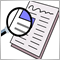Creating and Publishing of Trade Reports and SMS Notification

Traders don't always have ability and desire to seat at the trading terminal for hours. Especially, if trading system is more or less formalized and can automatically identify some of the market states. This article describes how to generate a report of trade results (using Expert Advisor, Indicator or Script) as HTML-file and upload it via FTP to WWW-server. We will also consider sending notification of trade events as SMS to mobile phone.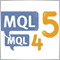Transferring Indicators from MQL4 to MQL5

This article is dedicated to peculiarities of transferring price constructions written in MQL4 to MQL5. To make the process of transferring indicator calculations from MQL4 to MQL5 easier, the mql4_2_mql5.mqh library of functions is suggested. Its usage is described on the basis of transferring of the MACD, Stochastic and RSI indicators.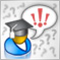Limitations and Verifications in Expert Advisors

Is it allowed to trade this symbol on Monday? Is there enough money to open position? How big is the loss if Stop Loss triggers? How to limit the number of pending orders? Was the trade operation executed at the current bar or at the previous one? If a trade robot cannot perform this kind of verifications, then any trade strategy can turn into a losing one. This article shows the examples of verifications that are useful in any Expert Advisor.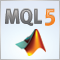MetaTrader 5 and MATLAB Interaction

This article covers the details of interaction between MetaTrader 5 and MatLab mathematical package. It shows the mechanism of data conversion, the process of developing a universal library to interact with MatLab desktop. It also covers the use of DLL generated by MatLab environment. This article is intended for experienced readers, who know C++ and MQL5.